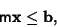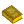## Polyhedron

A 3-D solid which consists of a collection of Polygons, usually joined at their Edges. The word derives from the Greek poly (many) plus the Indo-European hedron (seat). A polyhedron is the 3-D version of the more general Polytope, which can be defined on arbitrary dimensions.A Convex Polyhedron can be defined as the set of solutions to a system of linear inequalitieswhereis a realMatrix andis a real-Vector. An example is illustrated above. The more simple Dodecahedron is given by a system with. In general, given the Matrices, the Vertices (and Faces) can be found using Vertex Enumeration.

A polyhedron is said to be regular if its Faces and Vertex Figures are Regular (not necessarily Convex) polygons (Coxeter 1973, p. 16). Using this definition, there are a total of nine Regular Polyhedra, five being the Convex Platonic Solids and four being the Concave (stellated) Kepler-Poinsot Solids. However, the term regular polyhedra'' is sometimes also used to refer exclusively to the Platonic Solids (Cromwell 1997, p. 53). The Dual Polyhedra of the Platonic Solids are not new polyhedra, but are themselves Platonic Solids.

A Convex polyhedron is called Semiregular if its Faces have a similar arrangement of nonintersecting regular plane Convex polygons of two or more different types about each Vertex (Holden 1991, p. 41). These solids are more commonly called the Archimedean Solids, and there are 13 of them. The Dual Polyhedra of the Archimedean Solids are 13 new (and beautiful) solids, sometimes called the Catalan Solids.

A Quasiregular Polyhedron is the solid region interior to two Dual Regular Polyhedra (Coxeter 1973, pp. 17-20). There are only two Convex Quasiregular Polyhedra: the Cuboctahedron and Icosidodecahedron. There are also infinite families of Prisms and Antiprisms.

There exist exactly 92 Convex Polyhedra with Regular Polygonal faces (and not necessary equivalent vertices). They are known as the Johnson Solids. Polyhedra with identical Vertices related by a symmetry operation are known as Uniform Polyhedra. There are 75 such polyhedra in which only two faces may meet at an Edge, and 76 in which any Even number of faces may meet. Of these, 37 were discovered by Badoureau in 1881 and 12 by Coxeter and Miller ca. 1930.

Polyhedra can be superposed on each other (with the sides allowed to pass through each other) to yield additional Polyhedron Compounds. Those made from Regular Polyhedra have symmetries which are especially aesthetically pleasing. The graphs corresponding to polyhedra skeletons are called Schlegel Graphs.

Behnke et al. (1974) have determined the symmetry groups of all polyhedra symmetric with respect to their Vertices.

See also Acoptic Polyhedron, Apeirogon, Archimedean Solid, Canonical Polyhedron, Catalan Solid, Cube, Dice, Digon, Dodecahedron, Dual Polyhedron, Echidnahedron, Flexible Polyhedron, Hexahedron, Hyperbolic Polyhedron, Icosahedron, Isohedron, Johnson Solid, Kepler-Poinsot Solid, Nolid, Octahedron, Petrie Polygon, Platonic Solid, Polyhedron Coloring, Polyhedron Compound, Prismatoid, Quadricorn, Quasiregular Polyhedron, Rigidity Theorem, Semiregular Polyhedron, Skeleton, Tetrahedron, Uniform Polyhedron, Zonohedron

ReferencesSolid Geometry

Ball, W. W. R. and Coxeter, H. S. M. Polyhedra.'' Ch. 5 in Mathematical Recreations and Essays, 13th ed. New York: Dover, pp. 130-161, 1987.

Behnke, H.; Bachman, F.; Fladt, K.; and Kunle, H. (Eds.). Fundamentals of Mathematics, Vol. 2: Geometry. Cambridge, MA: MIT Press, 1974.

Bulatov, V. Polyhedra Collection.'' http://www.physics.orst.edu/~bulatov/polyhedra/.

Coxeter, H. S. M. Regular Polytopes, 3rd ed. New York: Dover, 1973.

Critchlow, K. Order in Space: A Design Source Book. New York: Viking Press, 1970.

Cromwell, P. R. Polyhedra. New York: Cambridge University Press, 1997.

Cundy, H. and Rollett, A. Mathematical Models, 3rd ed. Stradbroke, England: Tarquin Pub., 1989.

Davie, T. Books and Articles about Polyhedra and Polytopes.'' http://www.dcs.st-andrews.ac.uk/~ad/mathrecs/polyhedra/polyhedrabooks.html.

Davie, T. The Regular (Platonic) and Semi-Regular (Archimedean) Solids.'' http://www.dcs.st-andrews.ac.uk/~ad/mathrecs/polyhedra/polyhedratopic.html.

Eppstein, D. Geometric Models.'' http://www.ics.uci.edu/~eppstein/junkyard/model.html.

Eppstein, D. Polyhedra and Polytopes.'' http://www.ics.uci.edu/~eppstein/junkyard/polytope.html.

Hart, G. W. Virtual Polyhedra.'' http://www.li.net/~george/virtual-polyhedra/vp.html.

Holden, A. Shapes, Space, and Symmetry. New York: Dover, 1991.

Lyusternik, L. A. Convex Figures and Polyhedra. New York: Dover, 1963.

Malkevitch, J. Milestones in the History of Polyhedra.'' In Shaping Space: A Polyhedral Approach (Ed. M. Senechal and G. Fleck). Boston, MA: Birkhäuser, pp. 80-92, 1988.

Miyazaki, K. An Adventure in Multidimensional Space: The Art and Geometry of Polygons, Polyhedra, and Polytopes. New York: Wiley, 1983.

Paeth, A. W. Exact Dihedral Metrics for Common Polyhedra.'' In Graphic Gems II (Ed. J. Arvo). New York: Academic Press, 1991.

Pappas, T. Crystals-Nature's Polyhedra.'' The Joy of Mathematics. San Carlos, CA: Wide World Publ./Tetra, pp. 38-39, 1989.

Pugh, A. Polyhedra: A Visual Approach. Berkeley: University of California Press, 1976.

Schaaf, W. L. Regular Polygons and Polyhedra.'' Ch. 3, §4 in A Bibliography of Recreational Mathematics. Washington, DC: National Council of Teachers of Math., pp. 57-60, 1978.

Virtual Image. Polytopia I'' and Polytopia II'' CD-ROMs. http://ourworld.compuserve.com/homepages/vir_image/html/polytopiai.html and http://ourworld.compuserve.com/homepages/vir_image/html/polytopiaii.html.